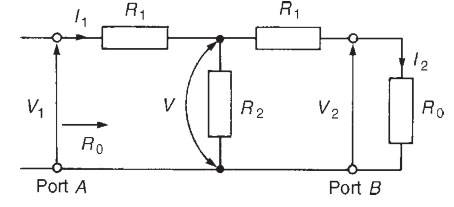# Insertion Loss in a (T) Two Port Network

agata78

## Homework Statement

Calculate the insertion loss for the network in the diagram?

## Homework Equations

20log(N)dB

N = R0 + R1 / R0 - R1

## The Attempt at a Solution

N = R0 + R1 / R0 - R1

N = 141.42 + 100 / 141.42 - 100

N = 241.42 / 41.42

N = 5.82858

20log(5.82858) = 15.311

Insertion Loss = 15.31 dB

Can someone please confirm I am correct or incorrect?

#### Attachments

•Skool.png
14.1 KB · Views: 710

Mentor

## Homework Statement

Calculate the insertion loss for the network in the diagram?

## Homework Equations

20log(N)dB

N = (R0 + R1) / (R0 - R1)

## The Attempt at a Solution

N = (R0 + R1) / (R0 - R1)

N = (141.42 + 100) / (141.42 - 100)

N = 241.42 / 41.42

N = 5.82858

20log(5.82858) = 15.311

Insertion Loss = 15.31 dB

Can someone please confirm I am correct or incorrect?

Looks good. You might use some parentheses to make the order of operations on your math clearYou probably should have explained where the R0 value came from, although it can be derived from the filter circuit itself with the assumption that it is impedance matched to the source and sink impedances.

shalteraR$_0{}$=$\sqrt{R^2{}_1{}+2R_{1}R_{2}}$

I suppose by 'N' you mean I1/I2

Last edited: